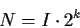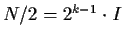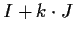Next: 2.1.7 Hybrid Representations of Up: 2.1.6 Alternate Representations of Previous: 2.1.6 Alternate Representations of

### 2.1.6.1 Arrays

Here is a sketch of how we might implement the BiList interface using arrays.

1.
newList: allocate a new array and initialize the elements in order (O(n))
2.
isEmpty: trivial if along with the array we maintain a count of how many elements are actually in use (O(1))
3.
insertFront: expensive. If the front is already occupied, we have to shuffle all the contents one place further down the array (O(n);
4.
insertRear: cheap (O(1));
5.
remRear: cheap (O(1));
6.
newIterator: we don't present an implementation, but the details are not hard. The iterator need only keep the index of the current element. But note that inserting or deleting from the middle now requires shuffling elements (O(n) average case).

If we run out of room we can resize the array used to store the list elements. If we double the size of the array at each resizing, then the average number of times an element is copied due to resizing is approximately 1. To prove this, let the initial size of the array be I, and suppose that the final size of the array is N, and there were k resizes. Thenand we observe that
1.
the first I elements move k times;
2.
the next I elements move k-1 times;
3.
the next 2I elements move k-2 times;
4.
...
5.
the lastelements move 0 = k-k times.

Using some summation facts we can show that the total number of array element copy operations is exactly N - I. Thus the average number of copy operations per element in the final array is (N-I)/N which is always less than 1, and approaches 1 in the limit as N gets much larger than I (i.e. as the number of resizings gets large). We say that the amortized cost of copying array elements is (bounded by a) constant. The strategy of doubling the size of the array on each resize operation appears to be an efficient one.

Exercise: Suppose that instead of doubling the array size, we increased it by some constant amount. That is, after kresizings, the size of the array isfor some constant J. What would the amortized cost of element copying be then?Next: 2.1.7 Hybrid Representations of Up: 2.1.6 Alternate Representations of Previous: 2.1.6 Alternate Representations of
Corky Cartwright
2000-01-07